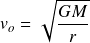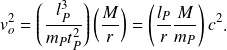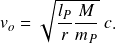# Orbital velocity

Orbital velocity tells us the strength of a gravitational field in terms of a second body orbiting a first. The formula quantifies the velocity at which the second body’s inertia is equal to the transverse gravitational field strength.

The gravitational field strength is determined by the mass density operator, which gives the ratio of length to mass in a region of space. Since the mass density operator acts on both parts of the 2-part energy mechanism (wavelength and velocity), the square root of the operator is taken.

## Historical formula

The elementary formula for orbital velocity is derived from the historical formula by replacing the gravitational constant with elementary units of length, mass, and time. The equationrestated in natural units isReducing the formula gives the elementary formula in terms of an operator acting on the maximum velocity potentialOrbital velocity